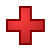Games
Problems
Go Pro!

Quizzes > Mathematics > Arithmetic > AdditionPractice your addition facts, adding single digit numbers. Students who remember these facts do their work much more efficiently than students who have to punch numbers into a calculator.

When you click Start Quiz, multiple-choice questions will appear one at a time. Choose the correct answers, and find out how much you know!
Quiz by Mr. TwitchellAssign this quiz# Blogs on This SiteReviews and book lists - books we love!The site administrator fields questions from visitors.

# Questions

Here are some of the questions you may encounter in the quiz Addition Facts (All).

9 + 1 = ?
1 + 2 = ?
5 + 0 = ?
9 + 2 = ?
3 + 4 = ?
8 + 0 = ?
5 + 7 = ?
1 + 9 = ?
8 + 9 = ?
8 + 6 = ?Like us on Facebook to get updates about new resources